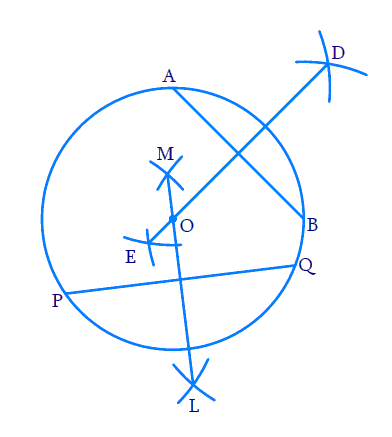# Ex.10.3 Q2 Circles Solution - NCERT Maths Class 9

Go back to  'Ex.10.3'

## Question

Suppose you are given a circle. Give a construction to find its center

Video Solution
Circles
Ex 10.3 | Question 2

## Text Solution

What is known?

A circle.

What is unknown?

Centre of the circle.

Reasoning:

By Theorem 10.3 (The perpendicular from the centre of a circle to a chord bisects the chord.) and by Theroem 10.4 (The line drawn through the centre of a circle to bisect a chord is perpendicular to the chord), we see that if a perpendicular bisector is drawn for a chord, it passes through the center. As it is not possible to find the center with the help of only one chord, two chords can be drawn. The intersection point of two perpendicular bisectors is the centre of the circle. Because the centre of the circle should lie on both the perpendicular bisectors of the two chords.

Steps:Construction:

Step 1: Draw a circle with convenient radius.

Step 2: Draw $$2$$ chords \begin{align}{AB} \end{align} and \begin{align}{PQ} \end{align} of any length.

Step 3: With \begin{align}{A} \end{align} as center and radii as more than half the length of \begin{align}{AB} \end{align}, draw two arcs on opposite sides of chord \begin{align}{AB} \end{align}. With the same radius and with \begin{align}{B} \end{align} as center, draw two arcs cutting the former arcs. Join the line. Now \begin{align}{DE} \end{align} is the perpendicular bisector of \begin{align}{AB} \end{align}. Centre lies on \begin{align}{DE} \end{align}.

Step 4: With $${P}$$ as center and radii as more than half the length of \begin{align}{PQ,} \end{align} draw two arcs on opposite sides of chord \begin{align}{PQ.} \end{align} With the same radius and with \begin{align}{Q,} \end{align} as center, draw two arcs cutting the former arcs. Join the line. Now $${LM}$$ is the perpendicular bisector of $${PQ}$$. Centre lies on $${LM}$$ also.

Step 5: As the center of the circle should lie both on $${DE}$$ and $${LM},$$ it is obvious that the intersection points of $${DE}$$ and $${LM}$$ is the center. Mark the intersection points as $${O}$$.

Step 6: $${O}$$ is the required centre of the circle.

Video Solution
Circles
Ex 10.3 | Question 2

Learn from the best math teachers and top your exams

• Live one on one classroom and doubt clearing
• Practice worksheets in and after class for conceptual clarity
• Personalized curriculum to keep up with school